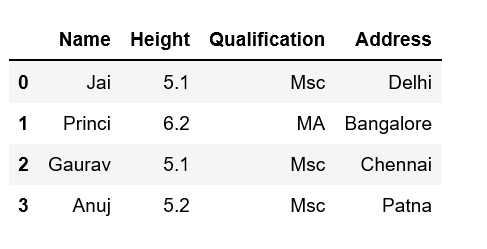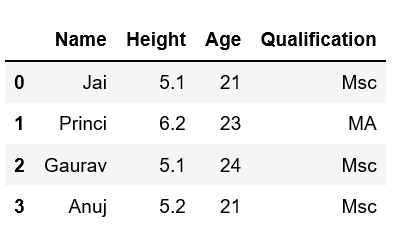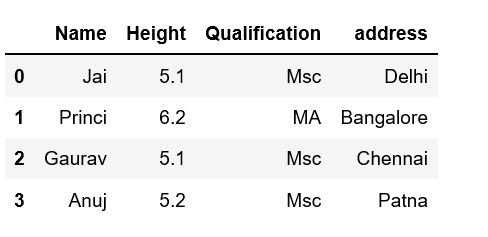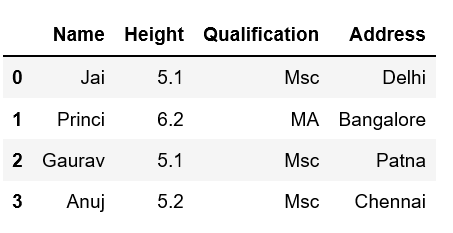GeeksforGeeks App
Open AppBrowser
Continue

# Adding new column to existing DataFrame in Pandas

Let’s discuss how to add new columns to the existing DataFrame in Pandas. There are multiple ways we can do this task.

Method #1: By declaring a new list as a column.

## Python3

 `# Import pandas package``import` `pandas as pd`` ` `# Define a dictionary containing Students data``data ``=` `{``'Name'``: [``'Jai'``, ``'Princi'``, ``'Gaurav'``, ``'Anuj'``],``        ``'Height'``: [``5.1``, ``6.2``, ``5.1``, ``5.2``],``        ``'Qualification'``: [``'Msc'``, ``'MA'``, ``'Msc'``, ``'Msc'``]}`` ` `# Convert the dictionary into DataFrame``df ``=` `pd.DataFrame(data)`` ` `# Declare a list that is to be converted into a column``address ``=` `[``'Delhi'``, ``'Bangalore'``, ``'Chennai'``, ``'Patna'``]`` ` `# Using 'Address' as the column name``# and equating it to the list``df[``'Address'``] ``=` `address`` ` `# Observe the result``print``(df)`

Output:Note that the length of your list should match the length of the index column otherwise it will show an error.

Method #2: By using DataFrame.insert()

It gives the freedom to add a column at any position we like and not just at the end. It also provides different options for inserting the column values.

Example

## Python3

 `# Import pandas package ``import` `pandas as pd`` ` `# Define a dictionary containing Students data``data ``=` `{``'Name'``: [``'Jai'``, ``'Princi'``, ``'Gaurav'``, ``'Anuj'``],``        ``'Height'``: [``5.1``, ``6.2``, ``5.1``, ``5.2``],``        ``'Qualification'``: [``'Msc'``, ``'MA'``, ``'Msc'``, ``'Msc'``]}`` ` `# Convert the dictionary into DataFrame``df ``=` `pd.DataFrame(data)`` ` `# Using DataFrame.insert() to add a column``df.insert(``2``, ``"Age"``, [``21``, ``23``, ``24``, ``21``], ``True``)`` ` `# Observe the result``print``(df)`

Output:Method #3: Using Dataframe.assign() method

This method will create a new dataframe with a new column added to the old dataframe.

Example

## Python3

 `# Import pandas package``import` `pandas as pd`` ` `# Define a dictionary containing Students data``data ``=` `{``'Name'``: [``'Jai'``, ``'Princi'``, ``'Gaurav'``, ``'Anuj'``],``        ``'Height'``: [``5.1``, ``6.2``, ``5.1``, ``5.2``],``        ``'Qualification'``: [``'Msc'``, ``'MA'``, ``'Msc'``, ``'Msc'``]}`` ` ` ` `# Convert the dictionary into DataFrame``df ``=` `pd.DataFrame(data)`` ` `# Using 'Address' as the column name and equating it to the list``df2 ``=` `df.assign(address``=``[``'Delhi'``, ``'Bangalore'``, ``'Chennai'``, ``'Patna'``])`` ` `# Observe the result``print``(df2)`

Output:Method #4: By using a dictionary

We can use a Python dictionary to add a new column in pandas DataFrame. Use an existing column as the key values and their respective values will be the values for a new column.

Example

## Python3

 `# Import pandas package``import` `pandas as pd`` ` `# Define a dictionary containing Students data``data ``=` `{``'Name'``: [``'Jai'``, ``'Princi'``, ``'Gaurav'``, ``'Anuj'``],``        ``'Height'``: [``5.1``, ``6.2``, ``5.1``, ``5.2``],``        ``'Qualification'``: [``'Msc'``, ``'MA'``, ``'Msc'``, ``'Msc'``]}`` ` `# Define a dictionary with key values of``# an existing column and their respective``# value pairs as the # values for our new column.``address ``=` `{``'Delhi'``: ``'Jai'``, ``'Bangalore'``: ``'Princi'``,``           ``'Patna'``: ``'Gaurav'``, ``'Chennai'``: ``'Anuj'``}`` ` `# Convert the dictionary into DataFrame``df ``=` `pd.DataFrame(data)`` ` `# Provide 'Address' as the column name``df[``'Address'``] ``=` `address`` ` `# Observe the output``print``(df)`

Output:My Personal Notes arrow_drop_up